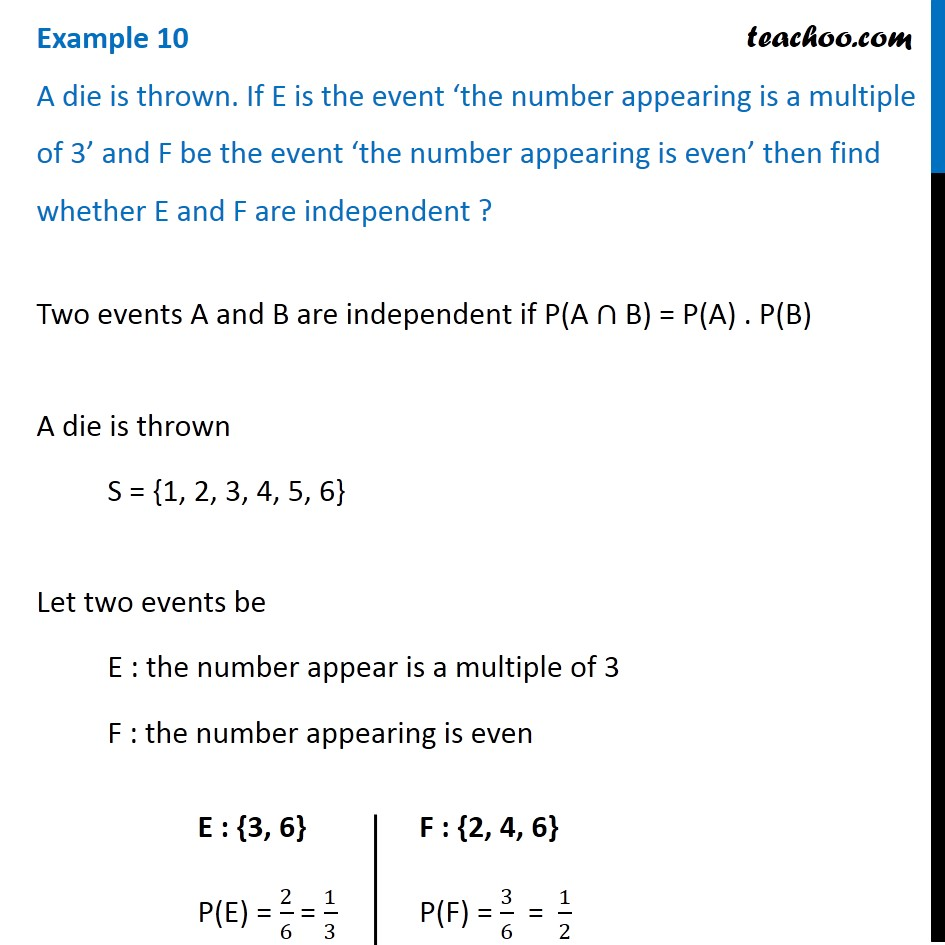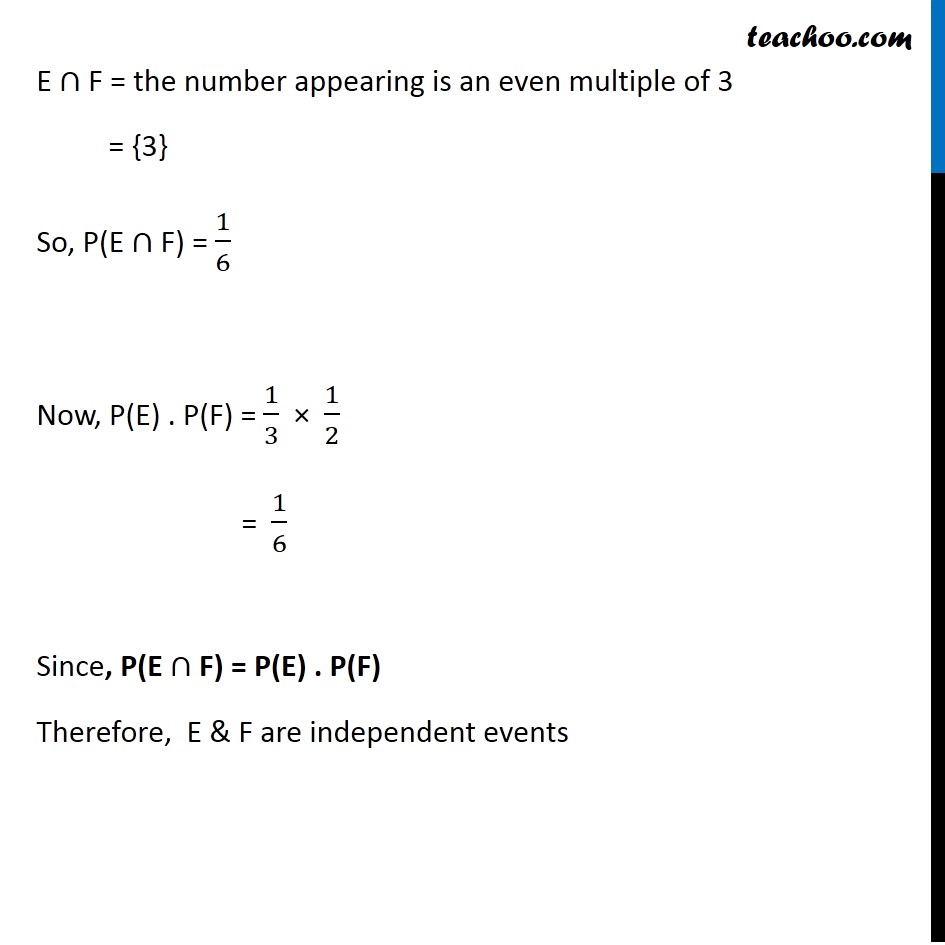Examples

Chapter 13 Class 12 Probability
Serial order wiseLearn in your speed, with individual attention - Teachoo Maths 1-on-1 Class

### Transcript

Example 10 A die is thrown. If E is the event ‘the number appearing is a multiple of 3’ and F be the event ‘the number appearing is even’ then find whether E and F are independent ? Two events A and B are independent if P(A ∩ B) = P(A) . P(B) A die is thrown S = {1, 2, 3, 4, 5, 6} Let two events be E : the number appear is a multiple of 3 F : the number appearing is even E : {3, 6} P(E) = 2/6 = 1/3 F : {2, 4, 6} P(F) = 3/6 = 1/2 E ∩ F = the number appearing is an even multiple of 3 = {3} So, P(E ∩ F) = 1/6 Now, P(E) . P(F) = 1/3 × 1/2 = 1/6 Since, P(E ∩ F) = P(E) . P(F) Therefore, E & F are independent events